##### Physics I For DummiesIn physics, how much torque you exert on an object depends on two things: the force you exert, F; and the lever arm. Also called the momentarm, the lever arm is the perpendicular distance from the pivot point to the point at which you exert your force and is related to the distance from the axis, r, by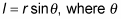is the angle between the force and a line from the axis to the point where the force is applied.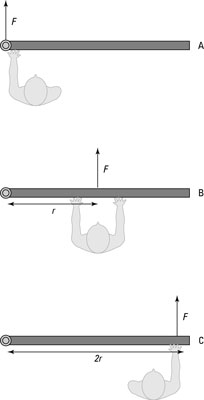The torque you exert on a door depends on where you push it.

Assume that you’re trying to open a door, as in the various scenarios in the figure. You know that if you push on the hinge, as in diagram A, the door won’t open; if you push the middle of the door, as in diagram B, the door will open; but if you push the edge of the door, as in diagram C, the door will open more easily.

In the figure, the lever arm, l, is distance r from the hinge to the point at which you exert your force. The torque is the product of the magnitude of the perpendicular force multiplied by the lever arm. It has a special symbol, the Greek letter tau: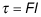The units of torque are force units multiplied by distance units, which are newton-meters in the MKS (meter-kilogram-second) system and foot-pounds in the foot-pound-second system.

For example, the lever arm in the figure is distance r (because this lever arm is perpendicular to the force), so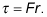If you push with a force of 200 newtons and r is 0.5 meters, what’s the torque you see in the figure? In diagram A, you push on the hinge, so your distance from the pivot point is zero, which means the lever arm is zero. Therefore, the magnitude of the torque is zero. In diagram B, you exert the 200 newtons of force at a distance of 0.5 meters perpendicular to the hinge, so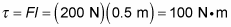The magnitude of the torque here is 100 newton-meters. But now take a look at diagram C. You push with 200 newtons of force at a distance of 2r perpendicular to the hinge, which makes the lever arm 2r or 1.0 meter, so you get this torque: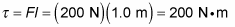Now you have 200 newton-meters of torque, because you push at a point twice as far away from the pivot point. In other words, you double the magnitude of your torque. But what would happen if, say, the door were partially open when you exerted your force? Well, you would calculate the torque easily, if you have lever-arm mastery.